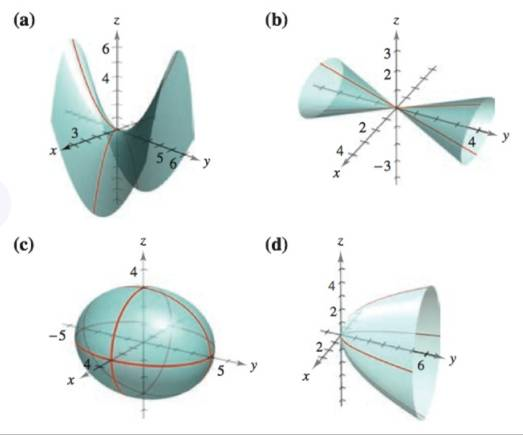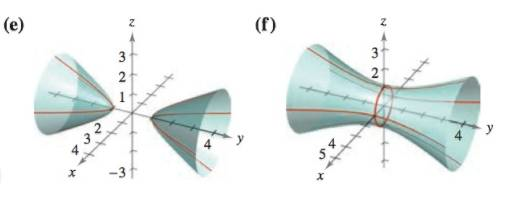Chapter 11.6, Problem 5E

Chapter
Section
Textbook Problem

# Matching In Exercises 5-10, match the equation with its graph. [The graphs are labeled (a), (b), (c), (d), (e), and (F).]4 x 2 − 4 y + z 2 = 0

To determine
Equation 4x24y+z2=0 is given. Find the graph that matches the given equation.

Explanation

Given:

Equation 4x24y+z2=0 is given.

Explanation:

Equation 4x24y+z2=0 is given.

simplify,

y=x2+z24 …… (1)

As we know elliptic paraboloid centered on y axis has a standard equation as mentioned below,

### Still sussing out bartleby?

Check out a sample textbook solution.

See a sample solution

#### The Solution to Your Study Problems

Bartleby provides explanations to thousands of textbook problems written by our experts, many with advanced degrees!

Get Started